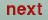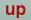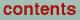Next: Token FORMAT= of cast Up: Category PLOT Previous: Tokens PMIN and PMAX=

### Tokens NDELR1, NDELR2, and NDELR3= of cast integer

Number of mesh points along the R1, R2, and R3 vectors respectively, or from RMIN to RMAX, from TMIN to TMAX, and PMIN to PMAX respectibely. If NDELR3=0 the charge density is evaluated in the plane spanned by R1 and R2. If NDELR2= is also 0 the charge density is evaluated along the line R1. For spherical coordinates NDELR1=0 gives plots on a sphere.

O. Jepsen
Thu Oct 12 14:48:45 MESZ 2000